Courses

# Notes | EduRev

## JEE Main Mock Test Series 2020 & Previous Year Papers

Created by: Learners Habitat

## JEE : Notes | EduRev

The document Notes | EduRev is a part of the JEE Course JEE Main Mock Test Series 2020 & Previous Year Papers.
All you need of JEE at this link: JEE

Rate of Reaction:

• Rate of change of extent of reaction is the rate of reaction.
• Rate of reaction is positive for product and negative for reactant.
• For reaction aA →bB
Rate =1/b(Δ[B]/ Δ t)  = -1/a (Δ [A]/ Δt)
• It goes on decreasing as the reaction progress due to decrease in the concentration(s) of the reactant(s).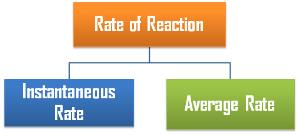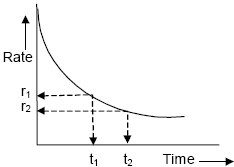• Unit of rate of reaction: mol L-1 s-1
• The rate measured over a long time interval is called average rate and the rate measured for an infinitesimally small time interval is called instantaneous rate.
• In a chemical change, reactants and products are involved. As the chemical reaction proceeds, the concentration of the reactants decreases, i.e., products are produced.
• The rate of reaction (average rate) is defined as the change of concentration of any one of its reactants (or products) per unit time.

Order of Reaction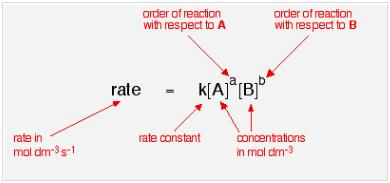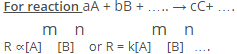Where m and n may or may not be equal to a & b.
m is order of reaction with respect to A and n is the order of reaction with respect to B.
m + n +… is the overall order of the reaction.

Elementary Reaction:

• It is the reaction which completes in a single step.
• A reaction may involve more than one elementary reactions or steps also.
• Overall rate of reaction depends on the slowest elementary step and thus it is known as rate determining step.

Molecularity of Reaction:

• Number of molecules taking part in an elementary step is known as its molecularity.
• Order of an elementary reaction is always equal to its molecularity.
• Elementary reactions with molecularity greater than three are not known because collisions in which more than three particles come together simultaneously are rare.
 Chemical Reaction Molecularity PCl5  → PCl3 + Cl2 Unimolecular 2HI  → H2 + I2 Bimolecular 2SO2 + O2  → 2SO3 Trimolecular NO + O3  → NO2 + O2 Bimolecular 2CO + O2  →  2CO2 Trimolecular 2FeCl3 + SnCl2 → SnCl2 + 2FeCl2 Trimolecular

Differential and Integrated Rate Laws:
Zero Order Reactions:
For Reaction: A → Product
[A]0-[A] = k0t
Where,
[A]0 = Initial concentration of A
[A]t = Concentration of A at time t.
k0  =  Rate constant for zero order reaction.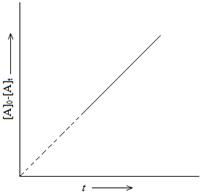Half Life:
t1/2 = [A]0/2k
Unit of rate constant = mol dm-3s-1

Examples:

•  Enzyme catalyzed reactions are zero order with respect to substrate concentration.
•  Decomposition of gases on the surface of metallic catalysts like decomposition of HI on gold surface.

First Order Reactions:
A → Product
(Δ [A] /A) = -k1Δt
or k1=( 2.303/ t)log ([A]0 / [A]t

Half Life:
t1/2 = 0.693/k1
Half life is independent of the initial concentration of the reactant for a first order
reaction.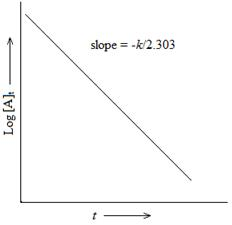Units of k=  s-1
Examples:
N2O5 →  2NO2 + 1/2O2
Br2 → 2Br
2HNO3 → 2NO + H2O
H2O2→ H2O + 1/2O2

Pseudo First Order Reactions:
These are the reactions in which more than one species is involved in the rate determining step but still the order of reaction is one.

Examples:

• Acid hydrolysis of ester: CH3COOEt + H3O→CH3COOH + EtOH
• Inversion of cane sugar: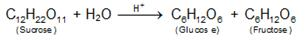• Decomposition of benzenediazonium halides C6H5N=NCl +H2O → C6H5OH +N2 +HCl

Half – Life of a nth Order Reaction:
kt1/2 =  (2n-1-1)/(n-1)[A0]n-1
Where, n = order of reaction ≠1

Parallel  Reactions:
The reactions in which a substance reacts or decomposes in more than one way are called parallel or side reactions.
If we assume that both of them are first order, we get.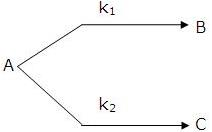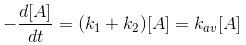k1 = fractional yield of B × kav
k2 = fractional yield of C × kav
If k1 >  k2 then
A → B main and
A → C is side reaction
Let after a definite interval x mol/litre of B and y mol/litre of C are formed.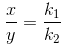i.e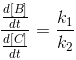This means that irrespective of how much time is elapsed, the ratio of concentration of B to that of C from the start (assuming no B  and C in the beginning ) is a constant equal to k1/k2.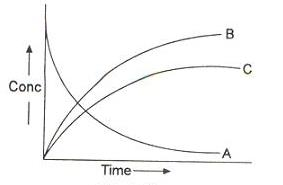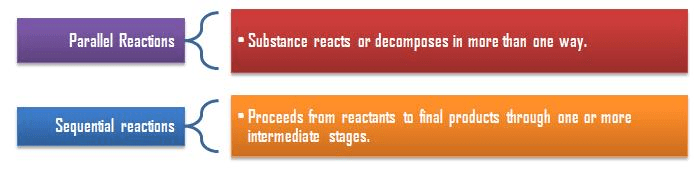Sequential Reactions:
This reaction is defined as that reaction which proceeds from reactants to final products through one or more intermediate stages. The overall reaction is a result of several successive or consecutive steps.
A → B → C and so on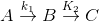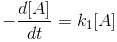…....(i)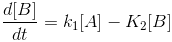…......(ii)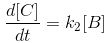….......(iii)
Integrating equation (i), we get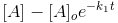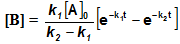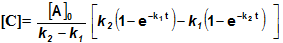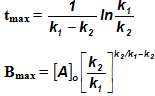Arrhenius Equation:
k = A exp(-Ea/RT)
Where, k = Rate constant
A = pre-exponential factor
Ea = Activation energy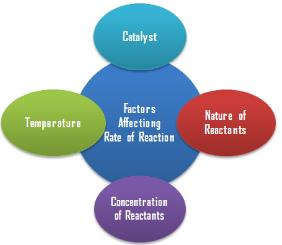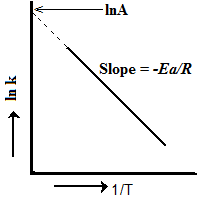Temperature Coefficient:
The temperature coefficient of a chemical reaction is defined as the ratio of the specific reaction rates of a reaction at two temperature differing by 10oC.
μ = Temperature coefficient= k(r+10)/kt
Let temperature coefficient of a reaction be ' μ ' when temperature is raised from Tto T2; then the ratio of rate constants or rate may be calculated as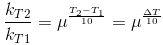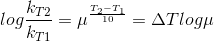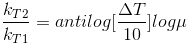Its value lies generally between 2 and 3.

Collision Theory of Reaction Rate

• A chemical reaction takes place due to collision among reactant molecules.
• The number of collisions taking place per second per unit volume of the reaction mixture is known as collision frequency (Z).
• The value of collision frequency is very high, of the order of 1025 to 1028 in case of binary collisions.
• Every collision does not bring a chemical change.
• The collisions that actually produce the products are effective collisions.
• The effective collisions which bring chemical change are few in comparison to the form a product are ineffective elastic collisions, i.e., molecules just collide and
• disperse in different directions with different velocities.
• For a collision to be effective, the following two barriers are to be cleared.
1. Energy Barrier
2. Orientation Barrier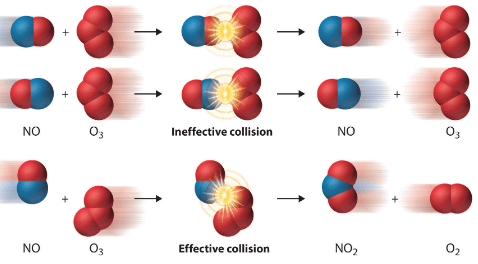-(dNA/dt) =l NA
Where, l =  decay constant of reaction
NA  = number of nuclei of the radioactive substance at the time when rate is calculated.
Arrhenius equation is not valid for radioactive decay.
Integrated Rate Law: N= Noe-lt
Half Life:  t1/2= 0.693/λ
Average life time: Life time of a single isolated nucleus, tav= 1/λ
Activity: Rate of decay
A = dNA/dt, Also, At = Aoe-lt
Specific Activity: activity per unit mass of the sample.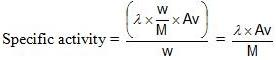Units: dps or Becquerrel

Offer running on EduRev: Apply code STAYHOME200 to get INR 200 off on our premium plan EduRev Infinity!

## JEE Main Mock Test Series 2020 & Previous Year Papers

3 videos|174 docs|149 tests

,

,

,

,

,

,

,

,

,

,

,

,

,

,

,

,

,

,

,

,

,

;